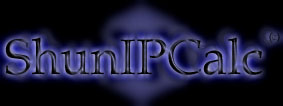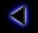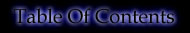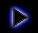.
What is a Subnet Mask ?
.
The Subnet Mask is deduced from the subnet structure (See "What is a subnet ?").
All bits corresponding to the Network and Subnet are "1"s and all bits corresponding to the Host are "0"s.
.
For example:
To the Class B IP: nnnnnnnn.nnnnnnnn.sssssshh.hhhhhhhh
Corresponds the subnet mask: 11111111.11111111.11111100.00000000
(where "n"s represent the Network, "s"s the Subnet and "h"s the Host)
.
This Subnet Mask in dotted decimal notation is 255.255.252.0. The "number of bits set to 1" notation is also commonly used. In this example, there are 22 "1"s so we write "/22".
.
..
Copyright (c) 2002 - Guillaume Péan
www.shunsoft.net